# Report by N.M Emanuel, 'Regarding the Nature of the Fallout Path of the Hydrogen Bomb Detonated on 1 March 1954 at Bikini [Atoll]'

This document was made possible with support from Carnegie Corporation of New York (CCNY)

A Report by N.M. Emanuel:

“Regarding the Nature of the fallout path of the Hydrogen Bomb detonated on 1 March 1954 at Bikini [Atoll].”

Official international sources (“A report by the United States Atomic Energy Commission on the effects of high-yield nuclear explosions” from 15 February 1955) as well as the press have recently given much attention to the question of the radioactive contamination created after the explosion of the hydrogen bomb at Bikini [Atoll] on 1 March 1954. These reports particularly emphasize the high levels of gamma radiation along the fallout path, and in relation to this, the dangers which may arise after the detonation of hydrogen bombs.

However, the information published in print is of interest for other reasons as well.  Such information makes an assessment possible of the nature of the exploding materials, and particularly—[how much fissile material was used in the bomb and how efficient the explosion was].

Assessments of such nature were made possible in 1954 when N.N Semenov, N.A Dmitriev, V.N Rodigin, and N.M Emanuel, produced a summary of the data on the radioactive contamination which was produced as a consequence of detonations of the RDS device at the UP-2 (see report [unclear] no. 58 for the year 1954). Consequently, the scaling of the radioactive fallout path formula, proposed by N.N Semenov, was evaluated. This formula allows us to assess the dose levels on the path for a device, if radiation levels for any path are known.

As is known, such dosage assessments assume great significance in the period before the conducting of new tests, and they provide a foundation for various practical activities regarding the provision of security for any humans and animals that may be found in the vicinity of the radioactive cloud’s path.

Thus, we set ourselves on a task to develop an assessment of the radioactive contamination which is to be expected following the 1 March 1954 explosion at Bikini [Atoll], if it would be possible to calculate it from the data on our own nuclear weapons tests. This assessment may be compared with the outcomes observed [in actuality] in the Bikini region.

How [does radioactive material] reach the fallout path? Primarily, due to fissile material from an atomic charge (either uranium or plutonium), which is utilized for the purpose of the initiation of a thermonuclear reaction.  It is necessary to take into consideration that part of the [radioactive material] was produced by the neutrons formed during a thermonuclear reaction.

Our report considers only that activity which was produced by [primary] fission fragments. Two cases were considered:

1. The activities on the path were produced by fission fragments which came about as a result of the primary.
2. We theoretically calculated the activity on the path that arose during the explosion of an atomic bomb of the same yield as the hydrogen bomb in Bikini (14 Megatons according to official data).

In both cases, the calculation was produced from the data on the radioactive path resulting from the surface blast at UP-2 in 1954 of a device that had a yield of 3 thousand tons [of TNT].

Moreover, an additional calculation is made on the basis of the data from the RDS-6 explosion. These calculations, as will be shown, point to an important principle about the nature of the fallout in the Bikini region, and enable us to articulate crucial inferences about the materials with which the hydrogen bomb was armed.

Reports written by the American Atomic Energy Commission on 15 February 1955 published the following data on levels of gamma radiation on the path:

 Distance from the explosion in kilometers Gamma radiation dosages 36 hours after the fall of radioactive particles in roentgens (R) 16 5000 160 2300 176 2000 200 1000 256 500 304 300

It is essential to know exactly when (how much time after the explosion) did the said particles reach a given location. This data can be obtained if the wind speed is known. In an article by R.I Len, published in the journal, “Bulletin of the Atomic Scientist,” II, 206,230 (1955), it is stated,  “… The amount of time which will be required for a radioactive cloud to travel over a distance of 110 miles (176 kilometers) from the location of the explosion, including the period of deposition of the radioactive particles, is five hours.”  This information allows us to find the value 176/5 = 35 km/h for the wind speed.

There are indications (see “Chemical and Engineering News,” [233?], 842 (1955),  and a report by the TACC news agency from April 2 1955) that on board of the Japanese ship “the Happy Dragon,” that was located roughly 70-80 miles from the explosion’s epicenter, “ash” began falling down three hours after the explosion. If we assume that the ship did not move too far from the center of the explosion during these three hours, then we can obtain wind speed at:

(70 × 1.6) ÷ (3) = 37 km/h, or (80 × 1.6) ÷ (3) = 43 km/h.

Our final value for the wind speed will be 35 km/h. The commission likewise published the radioactive path’s width over a distance of 140 miles (224 kilometers), which is 20 miles (32 km). Finally, it is possible to obtain the radioactive cloud’s height. After the explosion of the 5 Mt American hydrogen bomb in November 1952 [Ivy Mike], the cloud reached the height of about 20 kilometers. After the explosion of the RDS-6, the average height of the cloud was 12 kilometers.  Generally, for a device with high yield, where h > 10 km, height varies little as yield increases.  Thus, for a 14 Mt explosion, we can calculate the value 20 km for the cloud’s height.

Producing the mentioned above conversions allows us to make new and essential calculations. First, it is essential to use the dosage values, which are presented in the atomic commission’s report, for the purpose of obtaining the overall dosage levels from the moment of the explosion to infinity. This calculation can made with the following formula: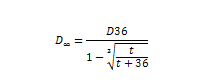In this formula Dand D36 – the general dosage and the dosage 36 hours after the explosion; t–the time at which the radioactive particles fell at the distances, where values are given for 36 hours .

After calculations on the basis of this formula we obtain the following table:

 Distance R in km D∞ in Roentgens 16 6500 160 4400 176 4000 200 2100 256 1110 304 715

Image 13 shows the dependency between D for the path of the hydrogen bomb’s detonated on 1 March 1954, and the [given] distance (on the double logarithmic scale). On the same image, on the same coordinates, the path is drawn for the 3,000 thousand ton atomic bomb which was detonated in 1954. Pay attention to the resemblance to the rule to which the change in general doses on the paths of the pure [fission] bomb (1954) and the hydrogen bomb (1 March 1954) is subordinate.Indeed, it is easy to see that the value of general doses on the path of the [fission] bomb decreases with the distance of the inversely proportional R2.5 (see report No.58 for 1954), and on the path of the hydrogen bomb- inversely proportional R3. We will remind that for the path of the RDS-6 device we received the law R3.1.

It is now possible to run the calculations which were discussed above.

First, based on the data on the path of the explosion of an atomic bomb with a yield of 3 thousand tons (1954) we will obtain the dimensions of the path which can be expected after an atomic bomb explosion that initiates a hydrogen bomb explosion. In an article by J. Rotblat (“Atomic Scientists Journal,” 4, 294 (1955)) it is stated that the [primary] of the hydrogen bomb has an explosive yield equaling 0.5 megatons, developing as a result of atomic fission chain reaction. We will calculate the gamma radiation dose which may be created by the detonation of the bomb’s primary In order to do this we will list here the characteristics of the 3 thousand ton atomic bomb explosion on UP-2 in 1954:

The energy of the explosion - E1= 3×103 ton TNT

Wind speed -                              V1= 34 km/h

The height of the cloud -    h1= 4 km.

The width of the path -              L1= 3–5 km, depending on the distance from explosion’s location.

For the calculation of the potential path produced by an explosion of a primary we will accept the following conditions:  E = 5×105 ton TNT, V=35 km/h; h=12 km;

For the width of the path and the height of the cloud’s rise we will take the same values that were observed after the explosion of the hydrogen bomb— that is, 32 km for a distance of [24?] km and a height of 20 km.

To convert, we will utilize the formula which was brought in the report of Semenov, Dmitriev, Rodigin, and Emanuel (inv no. 58 for the year 1954):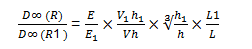In this formula D(R) and D (R1) mark the values of the general doses on the path, for the corresponding distances R and R1, which are related as such: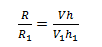Plugging into the conversion formula the numerical values for the explosion of the 3 thousand ton TNT device, as well as for the explosion of the primary we find that the relationship between the corresponding distances is: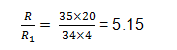The relationship of the general doses: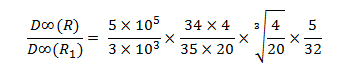Here the width of the path L1 is taken to be 5 km, [unclear] the exact same path width was observed for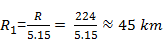(See report number 58 [unclear] for the year 1954).

The above calculation gives: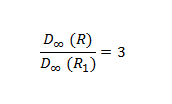The dependence of the general dose on the path on distance, calculated from these data, is shown in image 1 on the double logarithmic scale. It is not difficult to see that the path, which could have been produced by a 0.5 MT primary, would have been significantly smaller than the one actually observed after the explosion of a hydrogen bomb. For example, at a distance of 300 km from the detonation of the bomb, the general dose of gamma rays should have been 23 times smaller than what was actually observed.  [unclear] to assess a second calculation that is very important for us [unclear], the calculation of the path with the assumption that all of the power yielded after the explosion of the hydrogen bomb [unclear] energy released as a result of [unclear] of nuclear fuel.

All of the values needed for calculating are known to us:

E=14×106 ton TNT; V=35 km/h; h=20 km; L=32 km.

These are the same values we used in the preceding calculations, with the exception of the value of energy. In other words, we only need to increase all of the doses which were calculated for the explosion of the primary [by] [14×106]/[5×105] = 28 times, which is equivalent to the accepted value of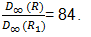The calculations for the path of a bomb with a yield of 14 Mt, assuming that all of the released energy was produced by the fission reaction, is presented on the dotted line. Comparing the calculated path with the one actually observed, we reach to the following immensely important conclusion:

The path which was calculated on basis of the assumption that the bomb was armed with fissile material almost completely matches observations.

This indicated that the hydrogen bomb, detonated on 1 March 1954 at Bikini [Atoll], contained a large quantity of fissile material, increasing the power of the atomic charge that is necessary for the initiation of a thermonuclear reaction.

In this context, it is necessary to reevaluate the reports coming from the international press about the “new type of a thermonuclear bomb,” and the “hydrogen-uranium bomb.”

In the article by J. Rotblat, cited earlier, it is confirmed that the activities produced by the explosion could be almost entirely attributed to fission fragments. It is also stated in the article that most of the energy produced by an explosion of a hydrogen bomb was produced by the fission process, and it is also stated that for this reason the hydrogen bomb is placed in a casing made of uranium-238. Neutrons separated during the thermonuclear reaction produce the fission of uranium [in the secondary casing].

The article’s author concludes that the hydrogen bomb detonated on 1 March 1954 in Bikini was a bomb with fission-fusion-fission reaction.

Finally, it is emphasized that if the bomb is based on a fission reaction, then radioactivity becomes the main factor to consider when evaluating the consequences of the explosion.  To these assertions by Rotblat we should add the information from the report by the ANSA agency from 14 June 1955. This report mentions the statement made by professor Libbi (from the American Atomic Energy Commission) who pointed to the existence of a bomb that is based on the fission principle with a yield of 10 megatons, the external casing of which contains uranium-238.

Finally, there are mentions in the report of events, observed after the explosion in Bikini, that were associated with the explosion of a hydrogen bomb of a new type.

As we saw, the calculations we made substantiate all of these assumptions. It is interesting to note that the 1 March 1954 explosion in Bikini is modeled [unclear] of the explosion of the RDS-6.

Indeed, in this case we should plug in the following numbers into the formula D (R)/ D (R1):

E1= 0.4 Mt; V1= 80 km/h; h1=12 km;

E=14 Mt; V= 34 km/h; h=20 km.

L=32 km over a distance of 224 km. As for the width of the path in the testing of the RDS-6 –the relationship between it and the distance is given in report inv. No. 58 for the year 1954. It is needed only to determine the distance which will correspond to 224 km.

To do this we will use the following ratios:

R/R1= Vh/V1 h1= (32x20)/ (80x12) = 0.71; R= 0.71R1,

That is, when R=224 km the value of R1 =315 km.

This value R1 will correspond to a path width of 32 km. In other words,

L1/L= 1.

A simple calculation gives,

D (R)/ D R1 = 41.6

Image 23 shows the ratios of the general doses on the RDS-6 path [unclear] and the [unclear] found by the formula.

We can see that the values obtained by way of calculations differ only by 1.5-2 times from the values actually observed (the calculated values are 2 times smaller than the observed values). Such deviations are not impossible.

Conclusions:

With the help of the ratio of similarity which enables us to make quantitative assessments of the doses on the path of the radioactive cloud IT IS SHOWN THAT THE HYDROGEN BOMB DETONATED IN BIKINI IN MARCH 1 1954 CONTAINED A LARGE QUANTITY OF FISSILE MATERIAL WHICH INCREASED THE POWER OF THE ATOMIC CHARGE NECESSARY FOR THE INITIATION OF A THERMONUCLEAR REACTION.

N.M Emanuel

24 August 1955.

Report by N.M. Emanuel on the U.S. Castle Bravo test in the Marshall Islands. The report surmises from fallout data that the bomb used a fission-fusion-fission design, and that the bomb contained a larger than normal amount of fissile material which induced a thermonuclear reaction.

## Document Information

### Source

Archive of the All-Russian Scientific Research Institute for Experimental Physics (VNIIEF), f. 1, op. 20s, ed. khr. 601, l. 1-12. Document no. 168 in Atomnyi proekt SSSR. Dokumenty i materialy. [The atomic project of the USSR. Documents and materials.], vol. 3, book 2, p. 368. Translated for CWIHP by Ronen Plechnin.

### Rights

The History and Public Policy Program welcomes reuse of Digital Archive materials for research and educational purposes. Some documents may be subject to copyright, which is retained by the rights holders in accordance with US and international copyright laws. When possible, rights holders have been contacted for permission to reproduce their materials.

2013-11-15

Report

118818

### Donors

Carnegie Corporation of New York (CCNY)